How To Do Test-Driven Development in Python (with Examples) - ByteScout
• Home
• /
• Blog
• /
• How To Do Test-Driven Development in Python (with Examples)

# How To Do Test-Driven Development in Python (with Examples)

Learning to code has always been a challenging thing to do. If you are a beginner, you can easily get overwhelmed with the types of development methodologies out there.

However, there is one method that you can utilize for better learning and development experience, i.e., Test-driven development(TDD).

Test-driven development(TDD) is one of the popular ways to code and create projects. It revolves around creating tests and then writing the actual code.

In this article, we will learn how to do test-driven development in Python. We chose Python because it is simple to learn and implement. However, before we get started with the actual tutorial, let’s explore TDD is detailed below.

## What is Test-Driven Development?

Test-driven development is a development philosophy where you need to check the code functionality before writing any “actual” code.

This process ensures that your code is as perfect as possible. With the tests code ready, you now know what needs to be included in your code to satisfy those tests. In TDD, major work is done during the test creation.

So, what steps are included in the TDD process? Let’s list them below.

• Write failing test
• Run and Fail Test
• Write Code To Pass
• Run and Pass Test
• Refactor

The above steps can be repeated until you get your intended result.

One more thing that you should be interested in knowing is that test-driven development works great with agile development. They both compliment each other as TDD results in faster development time.

Let’s list the benefits below.

• Improved code quality
• Faster development
• Clarity and focus
• Works with agile development
• Also, works with pair-programming

## How to do Test-driven development in Python(With Examples)

Before we get started, you need to make sure that you have Python installed on your computer. If you are sure, you are ready to proceed.

Create a parent folder. Inside that folder, you need to create two folders.

One folder is the app itself. Let’s name it calcapp and the other simply as “test.

Also, to make sure that you can import files from each of the folders, you need to create a file named, “__init__.py” in each of the directories.

Clearly, there are plenty of ways you can do test-driven development. But, for this tutorial, we are going to use the in-built unittest module. It comes pre-loaded with Python.

Now, you need to create a new file named test_calc.py.

For the tutorial, we are going to Windows. For the text editor, we will stick to Sublime Text.

In the tests.py file, we are going to create the following code.

import unittest

```class CreateFirstTest(unittest.TestCase):
def calculator_testing(self):
calcu = Calculator()
self.assertEqual(7, final)
```

As you can see, it is easy to create a test. Here we do the following things.

First, we import the unittest module. Next, we create a class for the test itself. Creating a Class can help you separate and manage your tests well.

Now, we define a method that initiates a calculator and does a sum. Lastly, we do a self.assertEqual() function.

To simplify our process of testing test cases, we are going to install nose. You can do it by running the following command.

pip install nose

Once done, it is easy to use.

To execute a single file, you can run the command below.

nosetests test_file.py

That means to make the tests.py file successfully you need to add the command below.

```if __name__ == '__main__':
unittest.main()
```

Let’s try to run the tests.py and see what it returns.

In the command prompt, you need to run the following command.

nosetests test_calc.py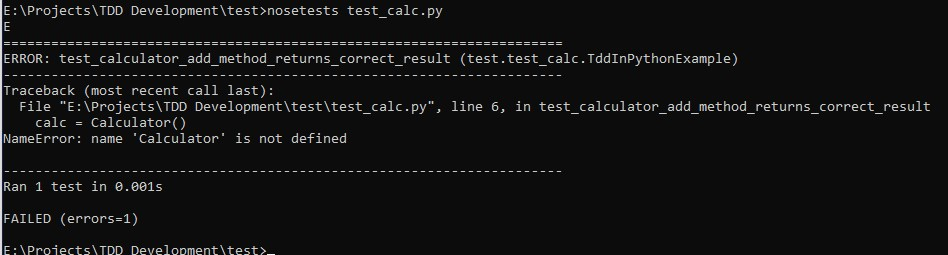As you can see that there is an error saying the Calculator is not defined. This is because of the fact that we have not defined and wrote code for the Calculator. Let’s create a file and name it, “calc.py”

Inside the file, you need to add the code as shown below.

```class Calculator(object):
pass
```

Now, go back to the tests.py and add the following line below “import unittest”

from calcapp.calc import Calculator

With everything set again, we can again run the nosetest.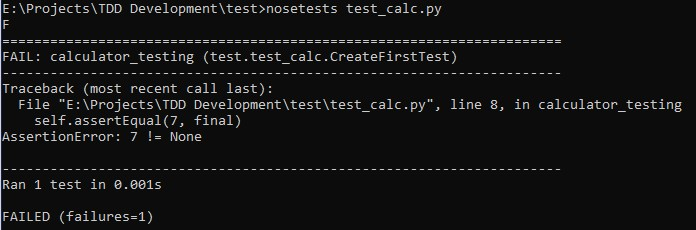From the above screenshot, you can see that it still fails the test. This is because we have not defined the add method yet.

To do so, copy the code below and add it to the calc.py file.

```class Calculator(object):
return a+b
```

Let’s do one more nosetest.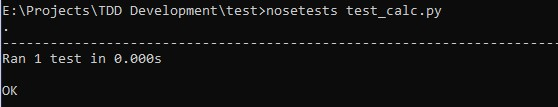It should successfully pass the test as shown above.

By now, you should now have a proper understanding of how test-driven development works. At the core, we simply need to satisfy the test cases. As a developer, your job is to create test cases for all possible scenarios. For instance, you should also take into consideration that the user can input strings instead of numbers.

Let’s try to create a test case for it.

```def test_if_input_is_not_number(self):
```

Here, we tried to use the assertRaises method to test the condition.

Also, we can move the calculator creation in the setUp method.

```def setUp():
self.calcu = Calculator()
```

This will help other methods to access the caclu variable.

If you run the nosetest now, it will give you the following error.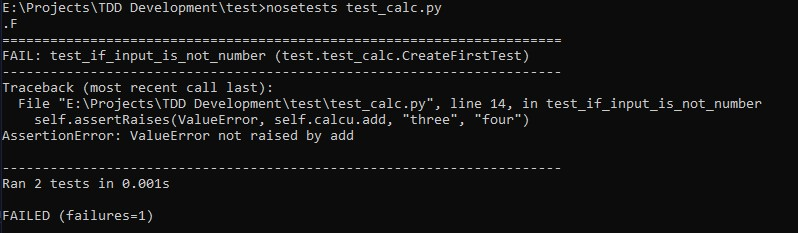To fix it, you need to add the types of input that the program will take from the user.

```class Calculator(object):
number_types = (int, float, complex)

if isinstance(a, number_types) and self.isinstance(b, number_types):
return a + b
else:
raise ValueError
```

Now, as you can see from the code above, we went forward and tried to check the instance of each of the inputs. If the instance matches the number type of int, long, float and complex, then we are going to do the addition and return the value. If the input is different than an integer, the function will simply return a ValueError.

To test all possible scenarios, we are going to add two more tests whereas one input is an integer and the other one is not.

The final code will be as below.

```import unittest
from calcapp.calc import Calculator

class CreateFirstTest(unittest.TestCase):
def setUp(self):
self.calcu = Calculator()

def calculator_testing(self):
self.assertEqual(7, final)

def test_if_input_is_not_number(self):

def test_first_input_not_number(self):

def test_second_input_not_number(self):

if __name__ == '__main__':
unittest.main()
```

Let’s try to run a final nosetest below.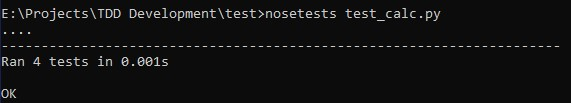We have successfully created a simple function of a calculator, i.e., addition. From here, you can decide to create the other functionalities of the calculator including subtraction, multiplication, and division. You may also go overboard and try to include high-level functionalities such as a log.

## Other Ways of Doing Test-Driven Development

Python is a versatile programming language that comes to options. In this section, we will briefly look at those options.

### UnitTest

Python’s unittest is the most popular package for creating and testing. If you want a nice output, then the unittest is a good option. It comes handy if you do not want to install an external package for testing. In our case, we used nosetest for testing.

To use unitttest, you need to simply add the following lines of code to your test file.

```if __name__ == '__main__':
unittest.main()
```

### py.test

Another option that you can use is the py.test. It is a test runner similar to the nosetest that we used in our tutorial. By using it, you will get the output in a separate area with nice details that can help you understand what went wrong.

To install it, you need to use the following command.

\$ pip install pytest.

Using it is similar to that of nosetest.

## Code Debugging Using Python Debugger

Not every time, you will find TDD to be as effective. As a programmer, you will find yourself stuck, unable to find what is the cause of the test failure.

That’s why you can use another approach where you can do code debugging. You can use the basic print statement in Python and utilize it to know the output at different stages of the program.

If that’s not what you are into, then you can make use of Python Debugger(PDB). It is very useful for debugging high-level code. We leave it to you to explore how to utilize it effectively in your programming.

## Conclusion

This leads us to the end of our test-driven development in Python. We hope that you now have a good understanding of TDD using Python. If you have any questions, then do comment below and let us know.ByteScout Team of Writers ByteScout has a team of professional writers proficient in different technical topics. We select the best writers to cover interesting and trending topics for our readers. We love developers and we hope our articles help you learn about programming and programmers.